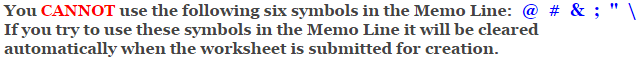# Calculus

## First Fundamental Theorem of Calculus Worksheets

These Calculus Worksheets will produce problems that involve using the first fundamental theorem of calculus to evaluate definite integrals.
The student will be given a definite integral and be asked to evaluate it by using the first fundamental theorem of calculus.
You may select the number of problems, and the types of functions.

These First Fundamental Theorem of Calculus Worksheets are a great resource for Definite Integration.

### Language for the First Fundamental Theorem of Calculus Worksheets

 English German Albanian Spanish Swedish Italian French Turkish Polish Norwegian

### Memo Line for the First Fundamental Theorem of Calculus Worksheets

You may enter a message or special instruction that will appear on the bottom left corner of the First Fundamental Theorem of Calculus Worksheets.### First Fundamental Theorem of Calculus Worksheets Answer Page

Now you are ready to create your First Fundamental Theorem of Calculus Worksheets by pressing the Create Button.

 Recommended Videos

If You Experience Display Problems with Your Math Worksheet